GRE Subject Test: Math : Binomial Distribution

Example Questions

Example Question #1 : Binomial Distribution

A fair coin is tossed 15 times. What is the probability of observing less than 3 heads?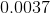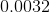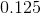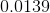Explanation:

This problem uses the Binomial Distribution: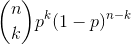For this problem n is the number of trials, or 15. Because the problem stated that the coin was a fair coin the probability of heads is one half, or .5.

The binomial distribution is a discrete distribution so the expression x<3 has to be broken down.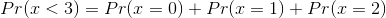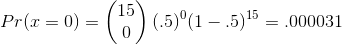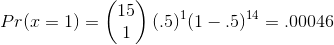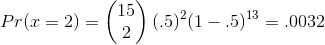Adding the probabilities will give the final answer.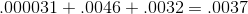All GRE Subject Test: Math Resources